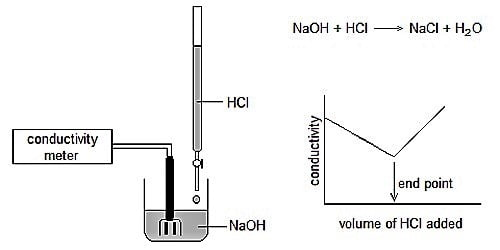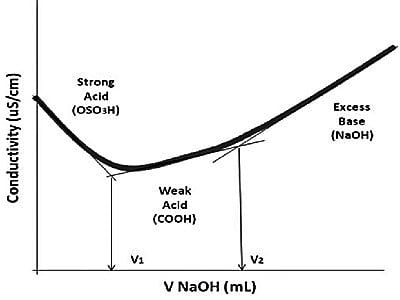Chemistry

# Advantages of Conductometric Titrations over Volumetric TitrationConductometric titration an exceptional case of volumetric analysis, it’s the method of measuring the endpoint of the titration. It is a volumetric process based on the measurement of conductance of the solution throughout the titration by the means of conductometer. The conductance of solution depends on the number and charge on free ions, the concentration of ions, temperature, and mobility of the ions. Few advantages of conductometric titration are: Conductometric titration can be used with much-diluted solutions and weak acids.Fig: Example of conductometric titration curve of protonated CNC-containing weak acid groups

Conductometric titrations have the following advantages over volumetric titration:

The main advantages to the conductometric titration are its applicability to very dilute and colored solutions and to a system that involves relative incomplete reactions. For example, which neither a potentiometric, nor indicator method can be used for the neutralization titration of phenol a conductometric endpoint can be successfully applied.

• Conductometric titration can be used with colored solutions where endpoint can’t be seen and can be used where there is no suitable indicator. No indicators are required and therefore, a colored solution can be conveniently titrated.  Conductometric titration can be accurately carved out for very dilute solutions, where indicator color change is not sharp.
• Conductometric titration can be used with many weak solutions and weak acids. This procedure is very functional in the titration of solutions that are very dilute, and even for very weak acids.
• For weak acid and weak base, no suitable indicators are available but conductometric titrations can be conveniently carried out.
• It gives more precise end-point. The end-point of this titration is very pointed and correct when compared to a few other titration processes.
• More accurate results are obtained by conductometric titrations because the end-point is determined graphically from a number of measurements and not from a single measurement as in volumetric titration.
• This titration can be used with colored or opaque solutions in which endpoint cannot be seen by the naked eye. This type of titration is appropriate for colored and turbid solutions for which the end point of titrations with usual indicators cannot be experiential simply with the human eye.
• Volumetric titration does not give correct endpoint for polybasic acids, while conductometric titration can be conveniently used in such cases.
• Conductometric titration can be used where there is no appropriate pointer. Since the end point is detected graphically, no enthusiastic examination is essential near the endpoint.
• The procedure of conductometric titration has many applications such as its use in acid-base titrations, redox titrations, complex titrations, and precipitation titrations.

However, it must be kept in mind that in order to minimize the volume change as little as possible the titer should be about 10 times stronger than the solution to be titrated. Also, the temperature of the solution should be kept constant during titration.

Conductometric titration is done by monitoring the electrolytic conductivity of a given reaction mixture while one reactant is constantly added to it. The theory of this type of titration states that the end-point consequent to the titration procedure can be indomitable by means of conductivity measurement. It is a laboratory system to spot the concentration of a given analyte in a substance. This theory can be used for either turbid or colored liquids in cases when you cannot distinguish the end-point by using indicators that are usual.

Types of conductometric titration:

• acid base titration,
• complexometric titration,
• replacement titration,
• redox titration,
• precipitation titration.